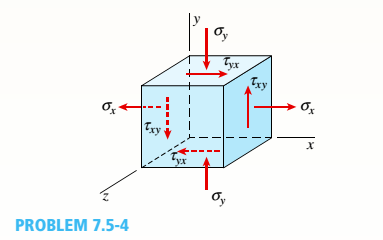# An element of a material is subjected to plane stresses as shown in the figure. The stresses o, cry., and are 10 MPa, —15 MPa, and 5 MPa. respectively. Assume E = 200 GPa and v = 0.3. (a) Calculate the normal strain in the x, v. and z directions and the shear strain. (b) Calculate the strain-energy density of the element.### Mechanics of Materials (MindTap Co...

9th Edition
Barry J. Goodno + 1 other
Publisher: Cengage Learning
ISBN: 9781337093347
Chapter 7, Problem 7.5.4P
Textbook Problem
1 views

## An element of a material is subjected to plane stresses as shown in the figure. The stresses o, cry., and are 10 MPa, —15 MPa, and 5 MPa. respectively. Assume E = 200 GPa and v = 0.3. (a) Calculate the normal strain in the x, v. and z directions and the shear strain. (b) Calculate the strain-energy density of the element.(a)

To determine

The normal strain in the x direction.

The normal strain in the y direction.

The normal strain in the z direction.

### Explanation of Solution

Given information:

The normal stress in the x direction is 10 MPa , the normal stress in y direction is 15 MPa , modulus of elasticity is 200 GPa , shear in the x y plane is 10 MPa, and the poison’s ratio is 0.3 .

Explanation:

Write the expression for the modulus of rigidity.

G = E 2 ( 1 + v ) ...... (I)

Here, the modulus of Rigidity is G , the shear modulus is E and Poisson’s ratio is v .

Write the expression for the strain in x direction.

ε x = 1 E ( σ x v σ y ) ...... (II)

Here, the normal stress in x direction is σ x , the normal stress in y direction is σ y , modulus of Elasticity is E and the Poisson’s ratio is v .

Write the expression for the strain in y direction.

ε y = 1 E ( σ y v σ x ) ...... (III)

Write the expression for strain in the z direction.

ε z = v E ( σ x + σ y ) ...... (IV)

Write the expression for the shear strain in x y plane.

γ x y = τ x y G ...... (V)

Here, the shear stress in the x y plane is τ x y and the shear modulus is G .

Calculation:

Substitute 10 MPa for σ x , 15 MPa for σ y , 200 GPa for E and 0.3 for v in Equation (I).

G = 200 GPa 2 ( 1 + 0.3 ) = 76.923 GPa

Substitute 10 MPa for σ x , 15 MPa for σ y , 200 GPa for E and 0.3 for v in Equation (II).

ε x = 1 200 GPa ( 10 MPa 0.3 ( 15 MPa ) ) = 1 200 GPa ( 10 9 Pa 1 Gpa ) ( 10 MPa ( 10 6 Pa 1 MPa ) 0.3 ( 15 MPa ( 10 6 Pa 1 MPa ) ) ) = 1 200 × 10 9 Pa ( 14.5 × 10 6 Pa ) = 7.25 × 10 5

Substitute 10 MPa for σ x , 15 MPa for σ y , 200 GPa for E and 0

(b)

To determine

The strain energy density of the element.

### Still sussing out bartleby?

Check out a sample textbook solution.

See a sample solution

#### The Solution to Your Study Problems

Bartleby provides explanations to thousands of textbook problems written by our experts, many with advanced degrees!

Get Started

Find more solutions based on key concepts
Explain how to use a push stick.

Precision Machining Technology (MindTap Course List)

Database Systems: Design, Implementation, & Management

In many applications, calibrated springs are commonly used to measure the magnitude of a force. Investigate how...

Engineering Fundamentals: An Introduction to Engineering (MindTap Course List)

5. Identify the main components of an information system. What is a mission-critical system?

Systems Analysis and Design (Shelly Cashman Series) (MindTap Course List)

The power supply converts the wall outlet AC power into DC power. (301)

Enhanced Discovering Computers 2017 (Shelly Cashman Series) (MindTap Course List)

What is the name of the eye burn that can occur in a fraction of a second?

Welding: Principles and Applications (MindTap Course List)

If your motherboard supports ECC DDR3 memory, can you substitute non-ECC DDR3 memory?

A+ Guide to Hardware (Standalone Book) (MindTap Course List)